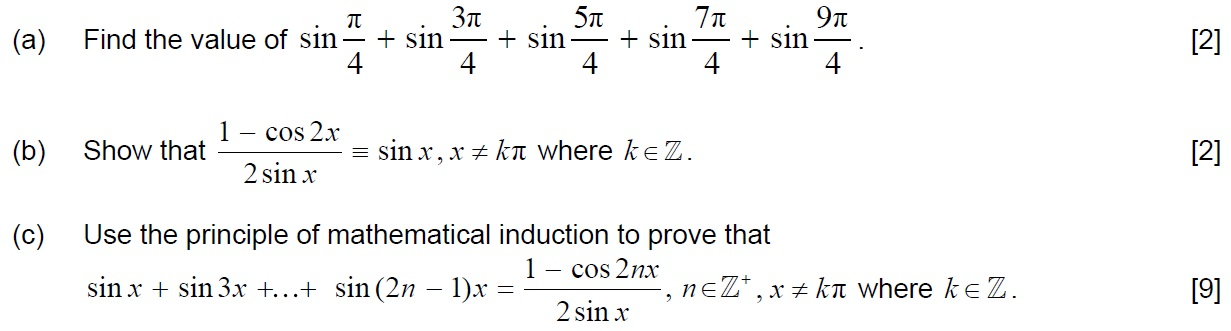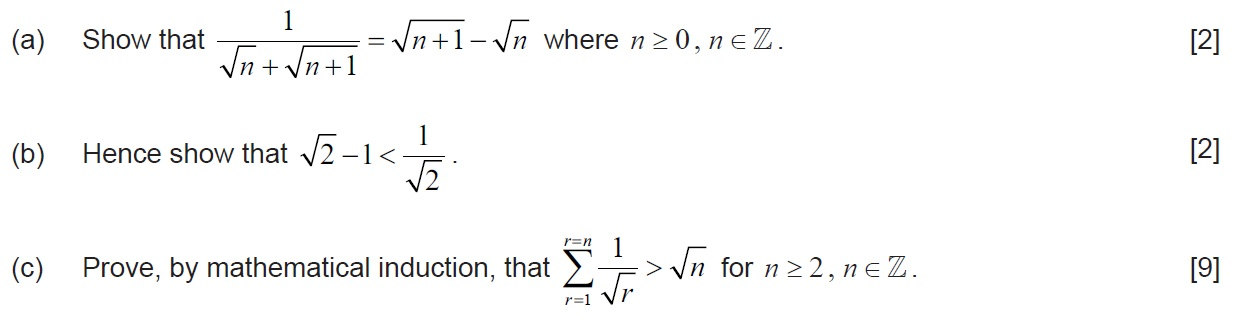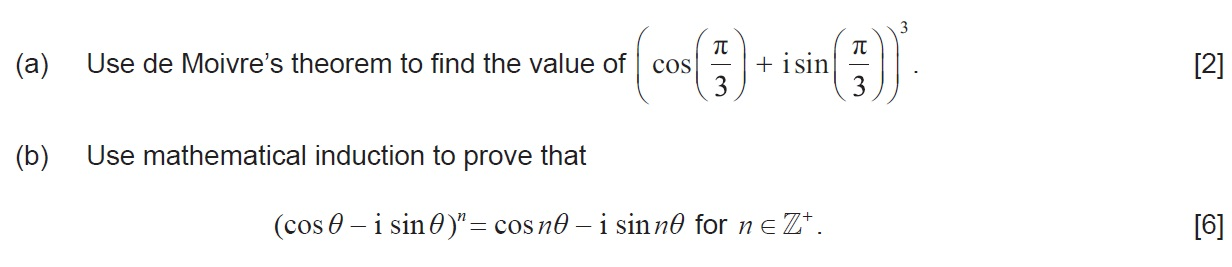# IBDP Past Year Exam Questions – Mathematical Induction

Q1.   [M09.P1.TZ2] & [N18.P1]

Prove by mathematical induction    .  

Q2.   [N09.P1]

Using mathematical induction, prove that  .                              .

Q3.   [M10.P1]

(a)   Consider the following sequence of equations.

$1×2=\frac{1}{3}\left(1×2×3\right)\phantom{\rule{0ex}{0ex}}1×2+2×3=\frac{1}{3}\left(2×3×4\right)\phantom{\rule{0ex}{0ex}}1×2+2×3+3×4=\frac{1}{3}\left(3×4×5\right)\phantom{\rule{0ex}{0ex}}...........$

(i)  Formulate a conjecture for the  ${n}^{th}$ equation in the sequence.

(ii)  Verify your conjecture for  $n=4$ .   

(b)       A sequence of numbers has the ${n}^{th}$ term given by  . Bill conjectures that all members of the sequence are prime numbers. Show that Bill’s conjecture is false. 

(c)        Use mathematical induction to prove that  $5×{7}^{n}+1$ is divisible by 6 for all  $n\in {\mathrm{ℤ}}^{+}$ .  

Q4.   [M08.P1]

Use mathematical induction to prove that for $n\in {\mathrm{ℤ}}^{+}$ ,

$a+ar+a{r}^{2}+.......+a{r}^{n–1}=\frac{a\left(1–{r}^{n}\right)}{1–r}$ .         

Q5.   [M11.P2] & [M18.P1]

Prove by mathematical induction that, for $n\in {\mathrm{ℤ}}^{+}$ ,

$1+2\left(\frac{1}{2}\right)+3{\left(\frac{1}{2}\right)}^{2}+4{\left(\frac{1}{2}\right)}^{3}+........+n{\left(\frac{1}{2}\right)}^{n–1}=4–\frac{n+2}{{2}^{n–1}}$ .   

Q6.   [M17.P1]

Use the method of mathematical induction to prove that ${4}^{n}+15n–1$ is divisible by  $9$ for  $n\in {\mathrm{ℤ}}^{+}$ .  

Q7.   [M13.P2]

Use the method of mathematical induction to prove that ${5}^{2n}–24n–1$ is divisible by $576$  for all  $n\in {\mathrm{ℤ}}^{+}$ . 

Q8.   [M14.P2]

Prove by mathematical induction that ${7}^{8n+3}+2$  ,  $n\in \mathrm{ℕ}$ ,  is divisible by $5$ . 

Q9.   [N16.P1]Q10.   [M15.P1]Q11.   [N14.P1]

Use mathematical induction to prove that  .   

Q12.  [M16.P1.TZ1]Q13.   [M10.P1]

(a)        Show that  $\mathrm{sin}2nx=\mathrm{sin}\left(\left(2n+1\right)x\right)\mathrm{cos}x–\mathrm{cos}\left(\left(2n+1\right)x\right)\mathrm{sin}x$ .

(b)        Hence prove, by induction, that

,

for all .

Q14.   [N17.P1]

Consider the function

(a)        Determine whether ${f}_{n}$ is an odd or even function, justify your answer.    

(b)        By using mathematical induction, prove that

where  $m\in \mathrm{ℤ}$ .